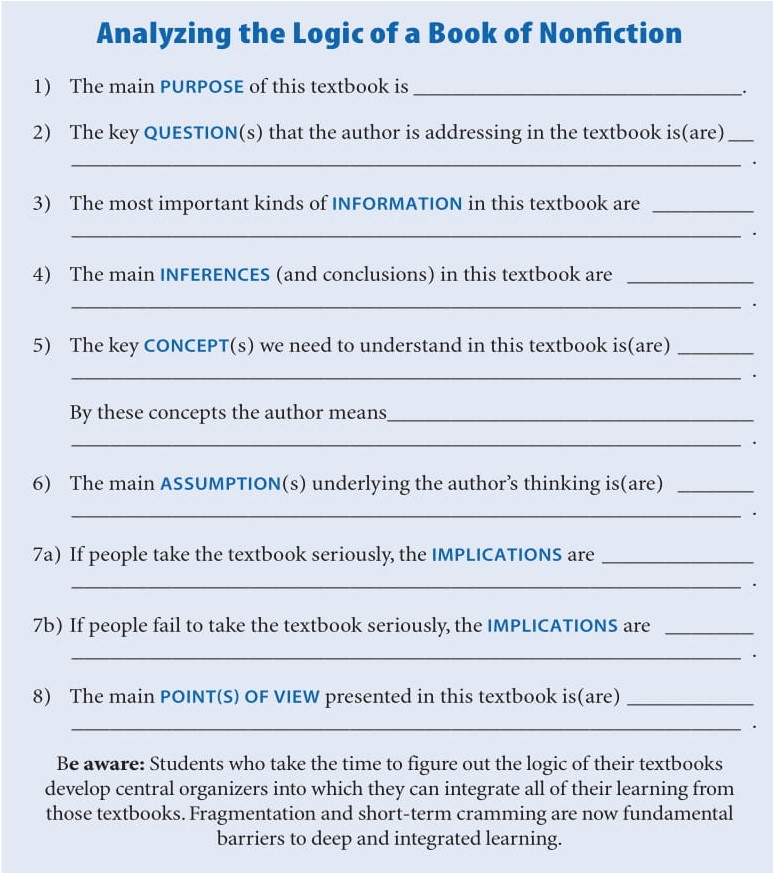Wheel of Reason Activity: Analyze the Logic of a Book of Nonfiction
Activity:
Analyze the Logic of a Book of Nonfiction
Below, we offer a template for analyzing the logic of a book of nonfiction. Here is the full template you should refer to when working through the logic of a book of nonfiction:Use this template for working through a book of nonfiction now:

The main purpose of this book of this textbook is:
The key question(s) the author is addressing in the textbook is(are):
The most important kinds of information in this textbook are:
The main inferences (and conclusions) in this textbook are:
The key concept(s) we need to understand in this textbook is(are):
By these concepts the author means:
The main assumption(s) underlying the author’s thinking is(are):
If people take the textbook seriously, the implications are:
If people fail to take the textbook seriously, the implications are:
The main point(s) of view presented in this textbook is(are):